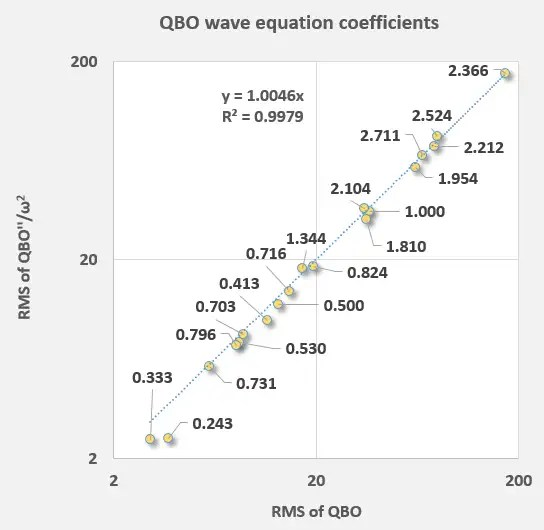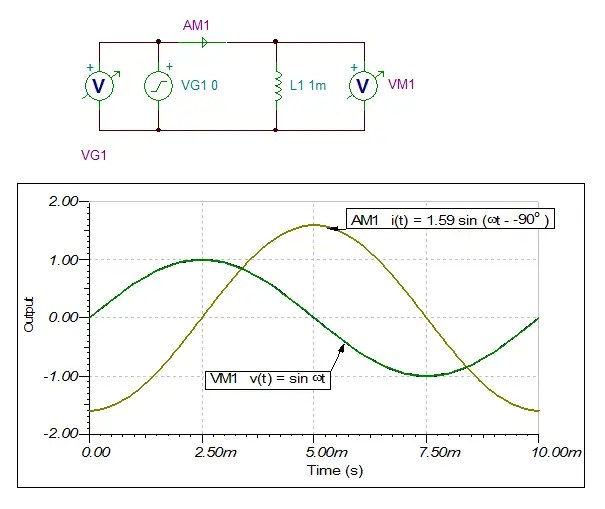# Inferring forced response from QBO wave equation

[mathjax]If the wave equation is to be effective in modeling a forced behavior such as QBO then certain invariant properties would need to be observed.$f''(t) + omega_0^2 f(t) = F(t)$

So if we split this into two parts$f''(t) approx k_1 F(t)$

and$omega_0^2 f(t) approx k_2 F(t)$

I have been applying multiple linear regression to fit the wave equation via either of the above two forms

So if we combine these two and see how well the Fourier series coefficients align — keeping care to compensate for the $$omega^2$$ scaling factor after taking the second derivative of a sinusoid — the linear result should be:$frac{f''(t)}{f(t)} = omega_0^2 k_1/k_2$

and indeed ! (see Figure 1 below)Fig 1: Correlation between direct f(t) and second-derivative f”(t) coefficient strengths. It should be linear with an intercept at the origin. The strongest term in the upper right is the aliased Draconic tidal period.  The rest are other lunar periods, solar periods, and aliased lunar harmonics.

The $$R^2$$ is 0.997

Remarkably, I did not apply any filtering to the QBO data, as each excursion is likely part of the signal — another substantiating check for the validity of this QBO model.

I’m looking for various arguments or analogies on how to get across a rather straight-forward idea — that a deterministic forcing response should be an acceptable alternative for modeling QBO.

In other disciplines, the forcing response is always considered first.

Say that we have an electrical circuit forced with a 100 Hz signal (could be 60 Hz but the time axis is easier to see with 100) . Figure 2 is a circuit featuring a single inductive element, with measured input voltage and the sensed output current.Fig 2:  The forcing period is invariantly matched from input to output, regardless of the complexity of the linear circuit.

Note the trivial result that the output is the same period as the input. This result isn’t dependent on the complexity of the circuit — as long as the transfer function is linear, the period will remain invariant. We can generalize this to multiple inputs with different periods (i.e. a Fourier series) and those same components will result, albeit with different amplitude and phase responses to each of the inputs.

So if we know the input periods, it is possible to use multiple linear regression to extract the amplitude and phase factors. And that is essentially what I did with the chart in Figure 1. The same idea can be applied to predicting ocean tides — the moon and the sun provides the forcing and the tidal gauge data gives the output.

The challenge that I face is to make a convincing argument that the QBO is simply a response to a similar periodic forcing, just as in the circuit diagram. I would have no problem if the circuit above was forced with a 60 Hz signal, and all we measured in the output was a 60 Hz signal. Another engineer would consider you stubborn if you refused to consider that the signal you were measuring was not from the AC line voltage.

Yet the reverse of that is what I am faced with in convincing others that QBO is the result of lunisolar tidal forcing. The main impediment is Richard Lindzen, who may have jumped the gun by ruling out that idea as early as 1968. To be generous, we can argue that he only had approximately 15 years of QBO data, whereas now we have another 50 years of data to substantiate the idea that the alias tidal periods are precisely the inputs. But Lindzen also had another 40 years to continue to compare his model with fresh data, so that he could have also validated his model’s correctness. Which he never apparently did **.

I am currently working on another set of QBO data. This consists of 14 time series at various altitudes. The initial results are self-consistent with the new model. Lindzen may have declared victory too early, while I will continue to search for flaws if I can.

** Lindzen may be need to be reminded of King Cnut, who proclaimed in the 12th century that trying to stop the tide would be a futile activity. This story is often twisted to portray that Cnut claimed he had the power to control nature, which kind of fits in with Lindzen’s ultimate failure to identify tidal forces. The tide may have rolled in and flattened his theory.